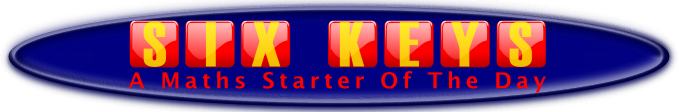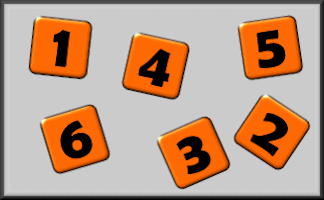#The challenge is to make 20 on your calculator using only six keys.

 4 7 - 2 7 = 20 5 + 7 + 8 = 20 1 2 0 ÷ 6 = 20 20

How many different ways can you do this? You could try making a different total. Is there a limit to the possible totals? What if you could use seven keys or five keys?

Share

Topics: Starter | Calculator

• Transum,
•
• Don't miss the opportunity for your pupils to access the pupil interactive version of this activity if they have computers, iPads or other internet access devices. The link to the activity can be found in large type below making it easy for them to copy it from the projected image of this page.
The pupil version records the solutions found by the pupils and they can copy and paste these solutions into their notes. The learning may be focussed on the number patterns and the sets of solutions found. The ultimate question of 'how many solutions are there?' should then become clearer.

How did you use this starter? Can you suggest how teachers could present or develop this resource? Do you have any comments? It is always useful to receive feedback and helps make this free resource even more useful for Maths teachers anywhere in the world.

If you don't have the time to provide feedback we'd really appreciate it if you could give this page a score! We are constantly improving and adding to these starters so it would be really helpful to know which ones are most useful. Simply click on a button below:

Excellent, I would like to see more like this
Good, achieved the results I required
Satisfactory
Didn't really capture the interest of the students
Not for me! I wouldn't use this type of activity.

This starter has scored a mean of 3.0 out of 5 based on 431 votes.

Previous Day | This starter is for 21 April | Next Day

 30 − 10 = 2031 − 11 = 2032 − 12 = 2033 − 13 = 2034 − 14 = 2035 − 15 = 2036 − 16 = 2037 − 17 = 2038 − 18 = 2039 − 19 = 2040 − 20 = 2041 − 21 = 2042 − 22 = 2043 − 23 = 2044 − 24 = 2045 − 25 = 2046 − 26 = 2047 − 27 = 2048 − 28 = 2049 − 29 = 2050 − 30 = 2051 − 31 = 2052 − 32 = 2053 − 33 = 2054 − 34 = 2055 − 35 = 2056 − 36 = 2057 − 37 = 2058 − 38 = 2059 − 39 = 2060 − 40 = 2061 − 41 = 2062 − 42 = 2063 − 43 = 2064 − 44 = 2065 − 45 = 2066 − 46 = 2067 − 47 = 2068 − 48 = 2069 − 49 = 2070 − 50 = 2071 − 51 = 2072 − 52 = 2073 − 53 = 2074 − 54 = 2075 − 55 = 2076 − 56 = 2077 − 57 = 2078 − 58 = 2079 − 59 = 2080 − 60 = 2081 − 61 = 2082 − 62 = 2083 − 63 = 2084 − 64 = 2085 − 65 = 2086 − 66 = 2087 − 67 = 2088 − 68 = 2089 − 69 = 2090 − 70 = 2091 − 71 = 2092 − 72 = 2093 − 73 = 2094 − 74 = 2095 − 75 = 2096 − 76 = 2097 − 77 = 2098 − 78 = 2099 − 79 = 20 10 + 10 = 20 100 ÷ 5 = 20 120 ÷ 6 = 20 140 ÷ 7 = 20 160 ÷ 8 = 20 180 ÷ 9 = 20 1 × 4 × 5 = 20 1 × 5 × 4 = 20 4 × 1 × 5 = 20 4 × 5 × 1 = 20 5 × 1 × 4 = 20 5 × 4 × 1 = 20 2 × 2 × 5 = 20 2 × 5 × 2 = 20 5 × 2 × 2 = 20 8 × 5 ÷ 2 = 20 5 ÷ 2 × 8 = 20 3 × 4 + 8 = 20 2 × 6 + 8 = 20 2 × 7 + 6 = 20 3 × 5 + 5 = 20 2 × 8 + 4 = 20 4 × 4 + 4 = 20 2 × 9 + 2 = 20 3 × 6 + 2 = 20 4 × 5 + 0 = 20 4 × 3 + 8 = 20 6 × 2 + 8 = 20 7 × 2 + 6 = 20 5 × 3 + 5 = 20 8 × 2 + 4 = 20 9 × 2 + 2 = 20 6 × 3 + 2 = 20 5 × 4 + 0 = 20 8 + 3 × 4 = 20 8 + 2 × 6 = 20 6 + 2 × 7 = 20 5 + 3 × 5 = 20 4 + 2 × 8 = 20 4 + 4 × 4 = 20 2 + 2 × 9 = 20 2 + 3 × 6 = 20 0 + 4 × 5 = 20 8 + 4 × 3 = 20 8 + 6 × 2 = 20 6 + 7 × 2 = 20 5 + 5 × 3 = 20 4 + 8 × 2 = 20 2 + 9 × 2 = 20 2 + 6 × 3 = 20 0 + 5 × 4 = 20 4 × 5 − 0 = 20 7 × 3 − 1 = 20 6 × 4 − 4 = 20 5 × 5 − 5 = 20 3 × 9 − 7 = 20 4 × 7 − 8 = 20 5 × 4 − 0 = 20 3 × 7 − 1 = 20 4 × 6 − 4 = 20 9 × 3 − 7 = 20 7 × 4 − 8 = 20

Your access to the majority of the Transum resources continues to be free but you can help support the continued growth of the website by doing your Amazon shopping using the links on this page. Below is an Amazon search box and some items I have chosen and recommend to get you started. As an Amazon Associate I earn a small amount from qualifying purchases which helps pay for the upkeep of this website.Teacher, do your students have access to computers?Do they have iPads or Laptops in Lessons? Whether your students each have a TabletPC, a Surface or a Mac, this activity lends itself to eLearning (Engaged Learning).Transum.org/go/?Start=April21

Here is the URL which will take them to a related student activity.

Transum.org/go/?to=6keysFor All: